New customer? Register here

 COVID-19 About Us AASHTO Accreditation Laboratory Assessments Proficiency Samples Falling Weight Deflectometer re:university Specifiers

The gold standard for laboratory accreditation in the construction industry

# Learn how to be an AASHTO lab 24/7

"“…if you do everything that the AASHTO accreditation requires you to do, if you execute all of the requirements of [AASHTO re:source], you will have a well-run laboratory."

Florida Department of Transportation

Tim Ruelke, P.E., Director, Office of Materials

 re:university Featured Document Library News & Announcements Newsletters Archives Video Gallery50+ years of experience 3,000+ PSP participants 23,000+ samples shipped per year 1,000 laboratory assessments per year 2,000+ accredited labs

# Math Matters: Dissecting Hydrometer Calculations

By Jonathan Sirianni, Senior Laboratory Assessor 2

Posted: April 2013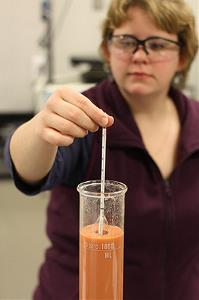In the 16th century Galileo and the Tower of Pisa made famous the fact that two objects, no matter their mass, fall at the same velocity. We know this to be true when dealing with objects in a vacuum. Drop a bowling ball and a feather inside a vacuumed tube, and they will reach the bottom of the tube at the same time. However, add air or water and the object’s mass and shape begin to factor largely into the speed with which an object will fall. Enter George Gabriel Stokes who, in the 1800s, discovered the mathematical formula for determining the speed at which spherical objects fall through a viscous fluid. Called Stokes’ Law, it essentially states that a more massive object will fall through a fluid more quickly than its less massive counterpart. It is this principle that is the foundation for Particle Size Analysis of Soils (AASHTO T 88 and ASTM D422).

The distribution of grain sizes within a soil sample helps in classifying the soil and determining its engineering properties. Based on Stokes’ Law, this procedure makes it possible to know the amount and size of the soil particles within a sample when the particles are too small to analyze using sieves. Larger soil particles will settle out of solution more rapidly than smaller particles. When using a sieve gradation, particle size can typically be determined down to 75 µm. However, with the help of a hydrometer, it is possible to establish particle sizes down to 1 µm. As particles settle, a density gradient is created. This can be quantified with the appropriate hydrometers and used to identify the properties of the particles within a suspension. This article focuses on the mathematics of the hydrometer portion of the test, and attempts to guide readers through the computation process.

Calculation Corner
The Particle Size Distribution of Soils Analysis standards contain complex calculations which are based on Stokes’ Law. Always remember that the calculations are based on spherical objects, though most soil particles will not truly be spherical in nature. This is for ease of use and, in general, it works well for the purpose of obtaining a gradation of the particles.

Hygroscopic Moisture:
Hygroscopic moisture is the natural moisture found in material due to water vapor naturally occurring in the air. Since the sample used for hydrometer testing is only air-dried, this type of moisture will still be present in the pores of the soil particles. To get an accurate estimation of grain size, it is imperative that an accurate sample mass is determined. AASHTO and ASTM each require hygroscopic moisture to be obtained from a fraction of the material passing a 2.00-mm (No. 10) sieve. The calculation is the same as any other moisture content taken during the soil testing process.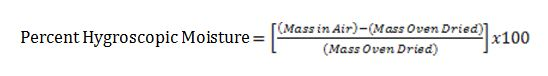With the percent hygroscopic moisture calculated, it is then possible to adjust the mass of the total sample to account for this moisture without the need to dry the entire sample in an oven and potentially damage the soil particles. To make this adjustment AASHTO makes use of the following equation: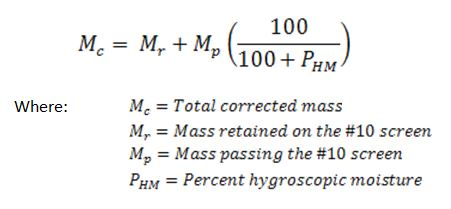This calculation makes the assumption that there is no natural moisture in the sample retained on the 2.00-mm (No. 10) sieve. In fact that is not the case, but due to the negligible amount of moisture within the pores of the larger sized particles, both AASHTO and ASTM require that the hygroscopic moisture correction only be applied to the material passing the 2.00-mm (No. 10) sieve (see note in AASHTO T 88). The total corrected mass (Mc) will then be employed for determining the percentages passing all sieves in the grain-size analysis. The ASTM version of the test does not include the equation above, but the calculations, which are described slightly differently, produce the same result.

Composite Correction:
The goal of a composite correction is to eliminate multiple variables with one procedure. First, sodium hexametaphosphate, used as a deflocculating agent during hydrometer testing, will change the inherent specific gravity of the suspension. It is imperative that this chemical is taken into account when taking hydrometer readings. Second, temperature variations during testing will also alter hydrometer readings because water density changes with temperature. Third, the cloudiness produced from the suspension of soil particles in the water will not allow the hydrometer to be read from the bottom of the meniscus. These three variables can skew the results of the test and produce inaccurate grain-size analyses. The composite correction will allow for all three variables to be removed from the measurements. Tables and graphs A and B show a typical composite correction.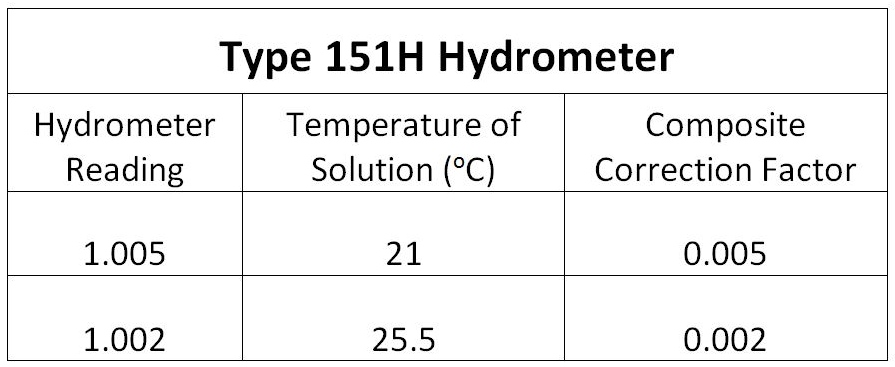Table A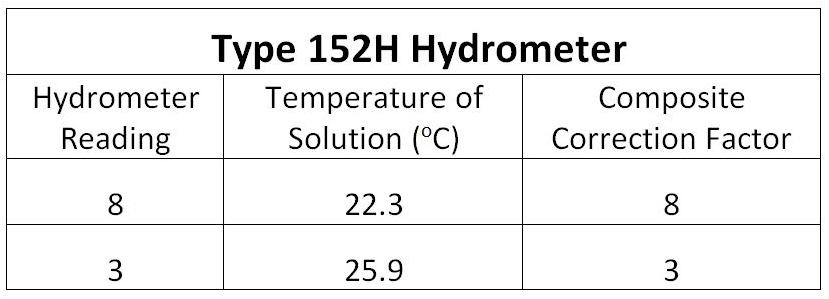Table B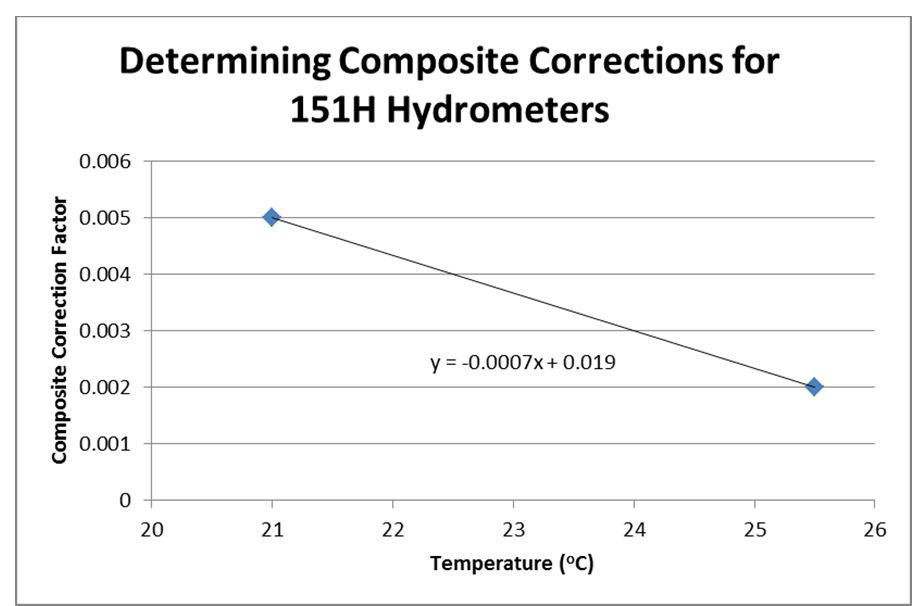Graph A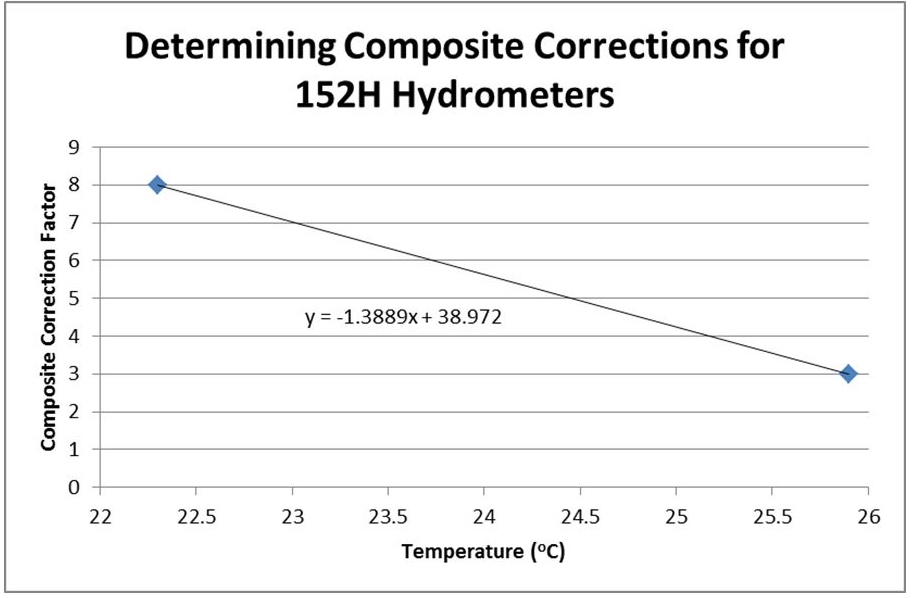Graph B

To obtain these results we only need to run a blank sample of 125 mL sodium hexametaphosphate solution (40 g/L) combined with 875 mL of distilled water (for a total volume of 1000 mL) at two temperatures. These two temperatures must encompass the expected values of temperatures that will occur during a normal grain-size analysis sedimentation test. Also it is important that the hydrometer readings are taken from the top of the meniscus. By utilizing the graph created for composite corrections, the line equation can be used to extrapolate the appropriate correction factor at any temperature during testing. To adjust the hydrometer reading with the correction factor, simply subtract the factors from the actual readings obtained throughout testing. Be sure to create a composite correction for each hydrometer being utilized for testing.

To illustrate using Graph B:

Line equation from graph:   y = -1.3889x + 38.972

y = Composite correction

x = Temperature (°C)

At 23.0°C we can find a composite correction factor by simply plugging in “23.0” to our equation.

y = -1.3889(23.0) +38.972

y = 7.0273

y = 7

This tells us that we will need to subtract “7” from any reading we obtain when testing soil suspensions at 23.0°C.

Key times to establish a new composite correction are when a new working solution of sodium hexametaphosphate is created (once a month), when employing a new hydrometer for use in testing, or if the expected temperatures during the particle size analysis will fall outside of the range used to create the initial composite correction.

Percentage of Soil in Suspension (P):
The percentage of soil in suspension calculation varies depending on the type of hydrometer used. The reasoning behind the differing calculations stems from what each hydrometer scale actually evaluates within the suspension. A type 151H hydrometer measures the specific gravity of the suspension. However, a type 152H hydrometer measures the density of solids in the suspension. To put it plainly, the numbers obtained from a 151H hydrometer are a comparison of the density of the suspension to the density of pure water at the same temperature. The numbers attained from the 152H hydrometer refer to the grams of solid material per liter of suspension.

If using an ASTM type 151H hydrometer the calculation for the percentage of soil in suspension differs slightly from one another when comparing the AASHTO and ASTM standards.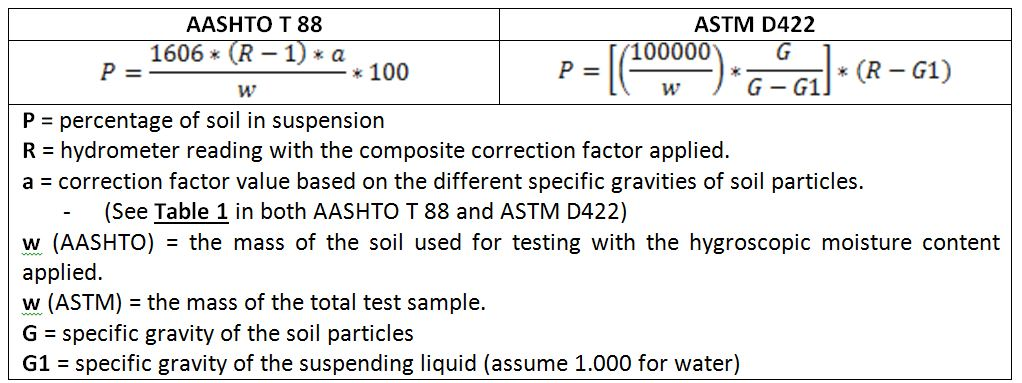Each of these two calculations will provide the same final percentage assuming all inputs are also equal. It is important to note that because the value used for w is slightly different in each standard, the result for in the AASHTO version of the equation must still be corrected by the percent retained on the 2.00-mm (No. 10) sieve (we will get back to this in the “Putting It All Together” portion of the article). On the other hand, the result for in the ASTM standard has already been corrected and does not need to go through this additional step. The example below will show that this is a case of both roads leading to the same final destination.

Example:

A solution consists of 96 g of moisture-corrected soil with a specific gravity of 2.65. The type 151H hydrometer reading indicates 1.019 after the composite correction is applied. What is the percentage of particles in solution?

If we plug in the numbers according to the individual standards we will obtain the following percentages.

AASHTO T 88: 31.785%          ASTM D422: 31.786%

But wait! Those are different numbers! Well not exactly. Due to significant digits, and because sample size controls the reportable resolution of test results, we can only report results to the nearest 0.1%. This means that AASHTO T 88 and ASTM D422 both will report our final percentage as being 31.8%. The negligible difference between the two equations is due to the fact that ASTM has assumed a portion of the equation to be constant, while AASHTO requires that the value be calculated based on the correction factor value,a.

If using an ASTM type 152H hydrometer the calculation for the percentage of soil in suspension is the same for both AASHTO T 88 and ASTM D422.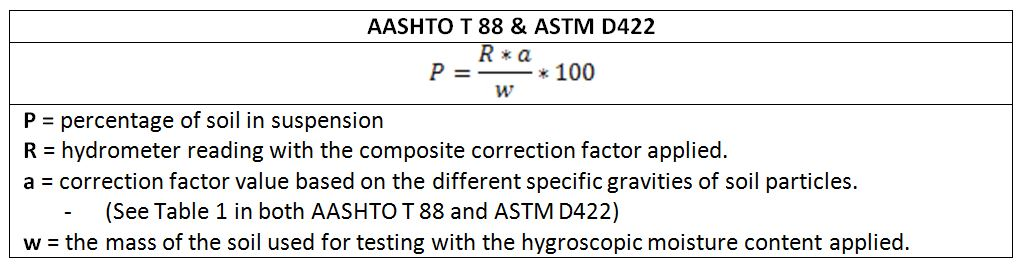If results from this calculation appear to make no sense, say having 120% of material in suspension, check to make sure the correct formula is being applied to the correct type of hydrometer.

Diameter of Soil Particles (D):
And now back to George Gabriel Stokes. Both AASHTO T 88 and ASTM D422 utilize Stokes’ Law for calculating the diameter of soil particles in suspension. Instead of going through the complex version of the calculation, both AASHTO and ASTM utilize an equation that is less daunting than the original concept.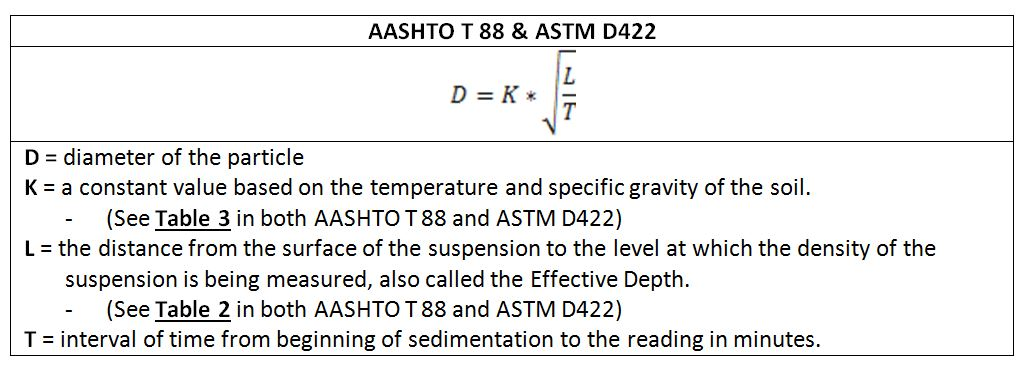Let’s take a quick a look at how AASHTO and ASTM arrived at this formula: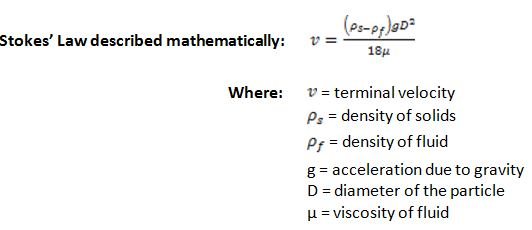Essentially this says that terminal velocity is directly related to the density of the soil, density and viscosity of the fluid that the soil is falling through, and the diameter of the particles. AASHTO and ASTM have simply reworked this formula to be a little more user-friendly and to solve for the particle diameter instead of terminal velocity. Table 2 and Table 3 in both standards provide shortcuts that eliminate the need to plug in many of the measurements that this original formula requires. Table 2 provides the effective reading depth (L) of the hydrometer. This is the length from the surface of the suspension to the hydrometer’s center of buoyancy. Essentially, it provides a means to know how far down the fluid column the particles have travelled. It is important to note that Table 2 in the ASTM version of the standard is in millimeters, while the same table in AASHTO lists values in centimeters. Table 3 supplies a constant (K) based on the matrix of soil density and temperature of the suspension. The constant takes all the density and viscosity variables and combines them into a single output number based on the assumption that the suspending fluid is water. Since velocity is the measure of length vs. time, all that needs to be done is to take the effective depth and divide it by the time of the measurement to establish the velocity of the particles within the suspension. By linking all the parts back together it is now possible to solve for the diameter of the particles. Because of the difference in units in Table 2, the values in the ASTM version of Table 3 must be divided by the square root of 10 to equal the values in the AASHTO version of Table 3.

Putting It All Together
The final piece of the puzzle requires us to take our percentage of particles in suspension (P) results and incorporate them with the total mass of the specimen. For AASHTO, the percentages that were found during the calculation procedures for hydrometer were based solely on the total material used for creating the hydrometer specimen. This means that, instead of being a percentage of total mass, it is only a percentage of what was originally placed into the hydrometer column. To make the hydrometer calculations useful, they need to be applied to the total mass, including the material retained on the 2.00-mm (No. 10) screen. This is only the case for the AASHTO version of the test. At this point (P) is already corrected to the total sample mass in the ASTM version of the test.After all of the hydrometer readings have been taken, the soil from the hydrometer column needs to be washed over a 75-µm (No. 200) sieve. The material retained on the 75-µm (No. 200) sieve should be dried back and sieved over a nest of sieves ranging from the 2.00-mm (No. 10) to the 75-µm (No. 200). This is an important step in the analysis because these relatively coarse materials tend to settle out of suspension within about 30 seconds and would not have been accounted for through hydrometer readings. The percentages obtained from this sieve analysis will also be only based the size of sample used for hydrometer testing and thus need to be calculated back to the total sample size.Graph C

Once all hydrometer calculations have been completed, the data can be graphed to give a visual representation of the total grain-size analysis. It is worth mentioning that the dry preparation method in ASTM requires that the plus 2.00-mm (No. 10) sieve material be washed, and the AASHTO dry preparation method does not. For this reason the ASTM version of the test may show slightly less material retained on the 2.00-mm (No. 10) sieve in the final results when the dry preparation method is used.

While this test method takes days to actually complete, it produces very useful information to assist in the classification of soils. Knowing the gradation of a soil beyond the 75-µm limitation of sieve analysis provides a better understanding of the physical properties associated with that sample.

New Grain-Size Analysis Test Methods for ASTM
ASTM is currently in the balloting process to separate the sieving and hydrometer portions of ASTM D422. To date they have released a new standard (ASTM D6913) that delves into a more efficient means of sieving the soil sample. AASHTO re:source now provides an on-site assessment option for this new test method. The hydrometer method is still in the balloting process and will follow as its own separate test method.

References

• AASHTO, “AASHTO T 88, Standard Method of Test for Particle Size Analysis of Soils,” Standard Specifications for Transportation Materials and Methods of Sampling and Testing, Part 2A: Tests, 2012.
• ASTM International, “ASTM D422, Standard Test Method for Particle-Size Analysis of Soils,” Book of Standards, Volume 04.08, 2007.
• Germaine, John T., and Amy V. Germaine, Geotechnical Laboratory Measurements for Engineers, Wiley, Hoboken, NJ, 2009.
• “Stokes’s Law,Encyclopedia Britannica, <http://www.britannica.com/EBchecked/topic/567002/Stokess-law> (February 1, 2013).

Printer Friendly Version

## 1 comment

1.asad | Jan 23, 2018